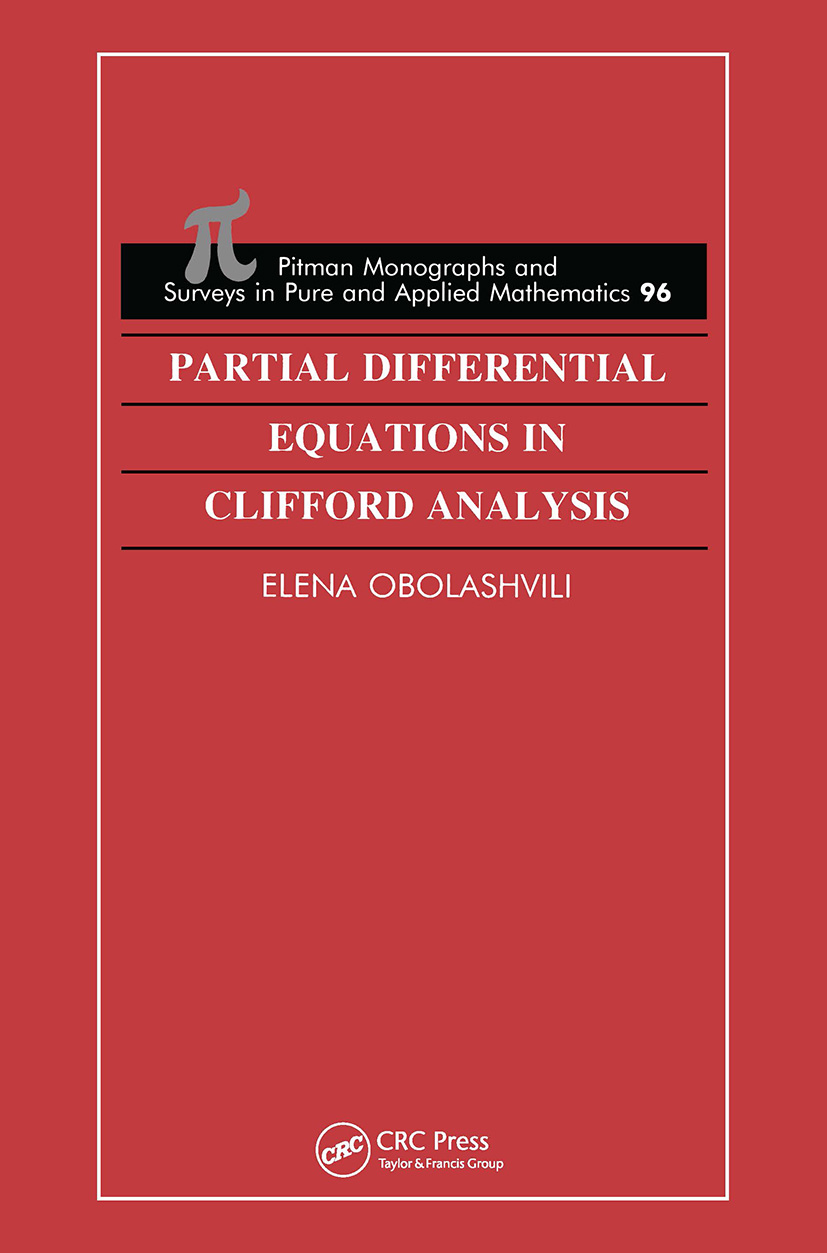# Partial Differential Equations in Clifford Analysis

## 1st Edition

Chapman and Hall/CRC

160 pages

##### Purchasing Options:\$ = USD
Hardback: 9780582317499
pub: 1999-01-06
Currently out of stock
\$185.00
x

FREE Standard Shipping!

### Description

Clifford analysis represents one of the most remarkable fields of modern mathematics. With the recent finding that almost all classical linear partial differential equations of mathematical physics can be set in the context of Clifford analysis-and that they can be obtained without applying any physical laws-it appears that Clifford analysis itself can suggest new equations or new generalizations of classical equations that may have some physical content.

Partial Differential Equations in Clifford Analysis considers-in a multidimensional space-elliptic, hyperbolic, and parabolic operators related to Helmholtz, Klein-Gordon, Maxwell, Dirac, and heat equations. The author addresses two kinds of parabolic operators, both related to the second-order parabolic equations whose principal parts are the Laplacian and d'Alembertian: an elliptic-type parabolic operator and a hyperbolic-type parabolic operator. She obtains explicit integral representations of solutions to various boundary and initial value problems and their properties and solves some two-dimensional and non-local problems.

Written for the specialist but accessible to non-specialists as well, Partial Differential Equations in Clifford Analysis presents new results, reformulations, refinements, and extensions of familiar material in a manner that allows the reader to feel and touch every formula and problem. Mathematicians and physicists interested in boundary and initial value problems, partial differential equations, and Clifford analysis will find this monograph a refreshing and insightful study that helps fill a void in the literature and in our knowledge.

Introduction

Principles of Clifford Algebra and Analysis

The Basic Notions and Definitions

Matrix Representations of Clifford Algebras for any Dimension

Differential Operators and Classification

Lorentz Transformations in the Elliptic and Hyperbolic Cases

Elliptic Partial Differential Equations

Introduction

Cauchy Kernel and Representations for h-regular Functions in R(n)

Extension Theorems and The Riemann-Schwarz Principle of Reflection

The Poincaré-Bertrand Transformation Formula

Generalized Riesz System

The Basic L-Theory of the Fourier Integral Transformation

Boundary Value Problems for Regular Functions with Values in R(n)

Boundary Value Problems for h-Regular Functions with Values in R(n), n³1

The Beltrami Equation in R(n)

Hyperbolic Partial Differential Equations

Introduction

Generalized Maxwell and Dirac Equations

The Hyperbolic Beltrami Equation

Initial Value Problems for the Klein-Gordon Equation

Cauchy's Initial Value Problem and its Modification for the Regular and h-Regular Functions with Values in R(n,n-1) and in R(n,n-2), n³3

Parabolic Partial Differential Equations

Introduction

Parabolic Regular System of the First Kind

Initial Value Problems for Parabolic Equations of the First Kind

Parabolic Regular Equations of the Second Kind and Initial Value Problems

Effective Solutions for Some Non-Local Problems

Introduction

Wiener-Hopf and Dual Integral Equations of Convolution Type

Generalized Wiener-Hopf Integral Equation with Two Kernels Depending on the Difference and Sum of the Arguments

Dual Integral Equations with Kernels Depending on the Difference and Sum of Arguments

Non-Local Problems for Holomorphic Functions and Applications in Elasticity Theory

Non-Local Problems for Generalized Holomorphic Functions and the Generalized Beltrami Equation

Non-Local Problems for Polyharmonic Functions

Epilogue

Bibliography

### Subject Categories

##### BISAC Subject Codes/Headings:
MAT007000
MATHEMATICS / Differential Equations
SCI040000
SCIENCE / Mathematical Physics